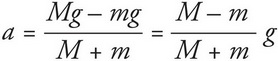# AP Physics 1 Question 206: Answer and Explanation

### Test Information

Question: 206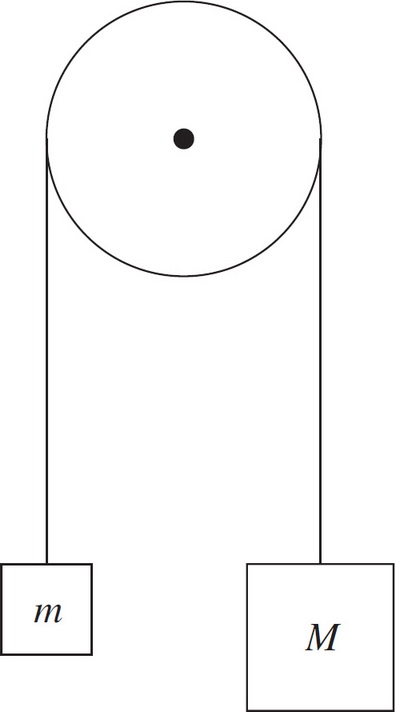6. Assuming a frictionless, massless pulley, determine the acceleration of the blocks once they are released from rest.

• A.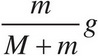• B.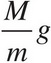• C.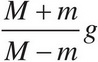• D.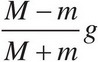Explanation:

D

One way to attack this question is to notice that if the two masses happen to be equal, that is, if M = m, then the blocks won't accelerate (because their weights balance). The only expression given that becomes zero when M = m is the one given in (D). Draw a free-body diagram: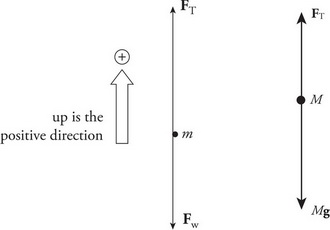Newton's Second Law gives the following two equations:

FT - mg = ma (1)

FT - Mg = M(-a) (2)

Subtracting these equations yields Mg - mg = ma + Ma = (M + m)a, so